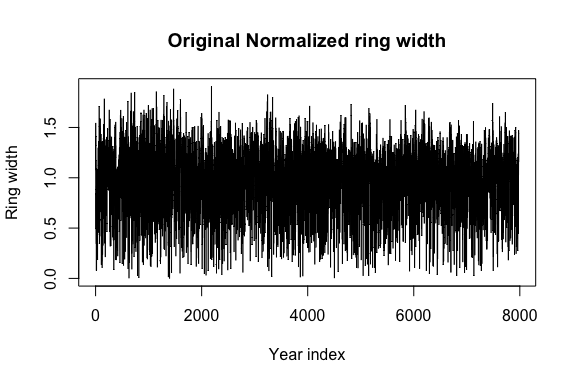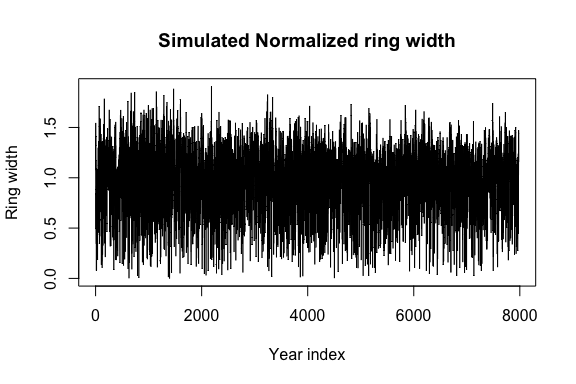# fakeR

## Motivation

As a response to concerns of anonymity and user privacy when releasing datasets for public use, fakeR is a package created to help allow users to simulate from an existing dataset. The package allows for simulating datasets of various variable types. This includes datasets containing categorical and quantitative variables as well as datasets of clustered time series observations. The package functions are also useful for maintaining a similar structure of missingness if one is to exist in the existing dataset.

One potential workflow for anonymization using this package would be to simulate fake data from the existing dataset to release to the public. From there, give others the opportunity to run analyses on the fake data and privately share their scripts to be rerun by the data owner on the real dataset. This procedure protects the anonymity of the individuals while allowing the analyses to be run on the real data for accurate end results. The amount of information from the original dataset to be shared in the simulated version can be specified, from approximate distribution .including covariances, between variables to the variable type only, with the data encoded with random numbers. Further research is currently being done to test and analyze such a method.

## Examples

### Simulate from time-independent data frame of multiple types

library(datasets)
library(fakeR)
library(stats)
# single column of an unordered, string factor
state_df <- data.frame(division=state.division)
# character variable
state_df$division <- as.character(state_df$division)
# numeric variable
state_df$area <- state.area # factor variable state_df$region <- state.region
state_sim <- simulate_dataset(state_df)
## Warning in data.class(current): NAs introduced by coercion
##  "Some unordered factors..."
##  "Numeric variables. No ordered factors..."

Notice how the function prints the variable types is notices while it is generating the simulated data.

head(state_df)
##             division   area region
## 1 East South Central  51609  South
## 2            Pacific 589757   West
## 3           Mountain 113909   West
## 4 West South Central  53104  South
## 5            Pacific 158693   West
## 6           Mountain 104247   West
head(state_sim)
##     area           division        region
## 1 110614    Middle Atlantic     Northeast
## 2  13687            Pacific          West
## 3 173203     South Atlantic         South
## 4 -10595           Mountain          West
## 5  83022           Mountain          West
## 6 101450 West North Central North Central

It is important to note that the multivariate normal assumption for generating numeric and ordered factor data is not always appropriate given the original data.

## Simulate from time-independent data frame with missingness & independence between variables

df <- mtcars
# change one of the variable types to an unordered factor
df$carb <- as.factor(df$carb)
# change another variable type to an ordered factor
df$gear <- as.ordered(as.factor(df$gear))
df[2,] <- NA
sim_df <- simulate_dataset(df, stealth.level=2, ignore='mpg', use.miss=TRUE)

### Simulate from time-dependent dataframe

## time series dataframe
tree_ring <- data.frame(treering)
tree_ring$year <- c(1: nrow(tree_ring)) sim_tree_ring <- simulate_dataset_ts(tree_ring, cluster="treering", time.variable="year") ##  "Some clustered time series data..." ##  "Processing done..." plot (tree_ring$year, tree_ring$treering, type='l', main=paste("Original","Normalized ring width"), ylab="Ring width", xlab="Year index")plot (tree_ring$year, tree_ring\$treering, type='l',
main=paste("Simulated","Normalized ring width"),
ylab="Ring width", xlab="Year index")Of note is the fact that the current options are to simulate data from a stationary or zero-inflated count time series.